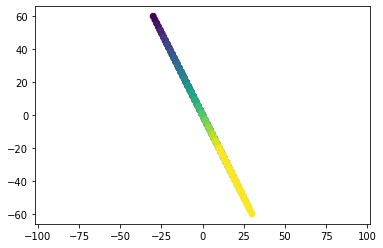In :
# General linear mapping
# A matrix A has two linearly dependent columns.

A4 = np.zeros((2,2))

A4[0,0] = 1
A4[0,1] = 2
A4[1,0] = -2
A4[1,1] = -4

X_new_mesh_4 = A4[0,0] * x_mesh + A4[0,1] * y_mesh
Y_new_mesh_4 = A4[1,0] * x_mesh + A4[1,1] * y_mesh

plt.scatter(X_new_mesh_4, Y_new_mesh_4, c=y_mesh)
plt.axis('equal')

Out:
(-33.009856630824366,
33.009856630824366,
-66.01517660044149,
66.01517660044149)